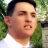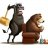# Get close for a specific time of the day

M

#### Max Grand

##### New member
Hi,
Does anybody know what is the best way to get the high/low of a bar in a 5-min aggregation time period?
I'm trying to compare 3 consecutive highs/lows in a 5-minute chart for running a scan out of it but the scan does not give any result...

Here's my code:

Code:
``````*********************
input ORBegin = 0930;

#First Bar
def ORActive1 = if SecondsTillTime(ORBegin+5*60) > 0 and SecondsFromTime(ORBegin) >= 0 then 1 else 0;
rec ORHigh1 = if ORActive1 == 1 then high else 0;
rec ORLow1 = if ORActive1 == 1 then low else 0;

#Second Bar
def ORActive2 = if SecondsTillTime(ORBegin+10*60) > 0 and SecondsFromTime(ORBegin+5*60) >= 0 then 1 else 0;
rec ORHigh2 = if ORActive2 == 1 then high else 0;
rec ORLow2 = if ORActive2 == 1 then low else 0;

#Third Bar
def ORActive3 = if SecondsTillTime(ORBegin+15*60) > 0 and SecondsFromTime(ORBegin+10*60) >= 0 then 1 else 0;
rec ORHigh3 = if ORActive3 == 1 then high else 0;
rec ORLow3 = if ORActive3 == 1 then low else 0;

def ThreeBarsUp = if ORHigh3 > ORHigh2 and ORHigh2 > ORHigh1 and ORLow3 > ORLow2 and ORLow2 > ORLow1 then 1 else 0;

plot Scan3BarsUp = ThreeBarsUp;``````
Any help is appreciated.

Max

Last edited:Close of 1 minute bar yesterday at specific time, show on today's 5 minute chart Questions 2Getting the open and close of the first 1 minute bar Questions 2